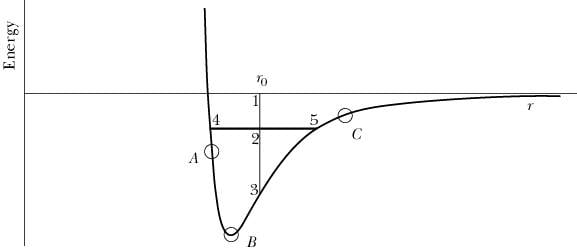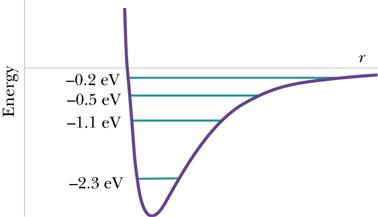# Potential Energy in a molecule

## Homework Statement

Potential energy in a molecule[/B](b) The figure below shows all of the quantized energies (bound states) for one of these molecules. The energy for each state is given on the graph, in electron-volts (1 eV = 1.6 ✕ 10-19 J). What is the minimum amount of energy required to break a molecule apart, if it is initially in the ground state? (Note that the final state must be an unbound state; the unbound states are not quantized.)
eV(c) If the temperature is high enough, in a collection of these molecules there will be at all times some molecules in each of these states, and light will be emitted. What are the energies in electron-volts of the emitted light? List them from smallest to greatest.

(d) The "inertial" mass of the molecule is the mass that appears in the definition of momentum, and through the momentum principle the inertial mass determines how much acceleration will result from applying a given force. Calculate the difference between the inertial mass of a molecule in the highest-energy bound state and the inertial mass of a molecule in the ground state.

## Homework Equations

D), delta_p=Fnet*delta_t
F X time = momentum
1 eV = 1.6e10-19J
E_N = -(13.6 eV)/N^2, where N is a nonzero positive integer
Photon energy and wavelength: E_photon = hc/gamma_light (h= plancks constant)

## The Attempt at a Solution

D), There should be no difference. The energy levels correspond to dierent types of energy not to a change in mass. I put 0 Kg but is incorrect. SO there is an actual change in MASS, but need help how to calculate it. Thanks!

Last edited by a moderator: